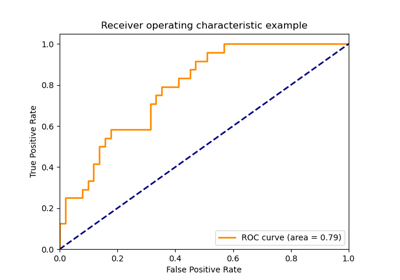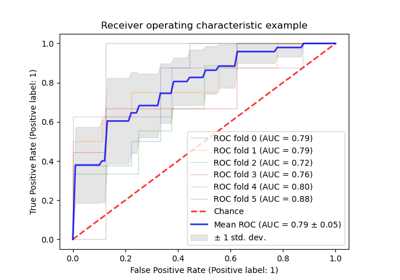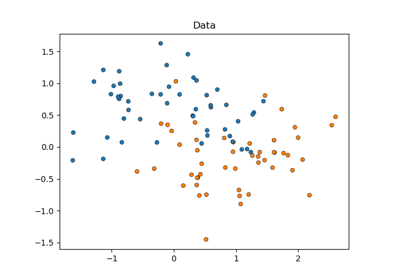# sklearn.metrics.roc_auc_score¶

sklearn.metrics.roc_auc_score(y_true, y_score, *, average='macro', sample_weight=None, max_fpr=None, multi_class='raise', labels=None)[source]

Compute Area Under the Receiver Operating Characteristic Curve (ROC AUC) from prediction scores.

Note: this implementation can be used with binary, multiclass and multilabel classification, but some restrictions apply (see Parameters).

Read more in the User Guide.

Parameters:
y_truearray-like of shape (n_samples,) or (n_samples, n_classes)

True labels or binary label indicators. The binary and multiclass cases expect labels with shape (n_samples,) while the multilabel case expects binary label indicators with shape (n_samples, n_classes).

y_scorearray-like of shape (n_samples,) or (n_samples, n_classes)

Target scores.

• In the binary case, it corresponds to an array of shape (n_samples,). Both probability estimates and non-thresholded decision values can be provided. The probability estimates correspond to the probability of the class with the greater label, i.e. estimator.classes_ and thus estimator.predict_proba(X, y)[:, 1]. The decision values corresponds to the output of estimator.decision_function(X, y). See more information in the User guide;

• In the multiclass case, it corresponds to an array of shape (n_samples, n_classes) of probability estimates provided by the predict_proba method. The probability estimates must sum to 1 across the possible classes. In addition, the order of the class scores must correspond to the order of labels, if provided, or else to the numerical or lexicographical order of the labels in y_true. See more information in the User guide;

• In the multilabel case, it corresponds to an array of shape (n_samples, n_classes). Probability estimates are provided by the predict_proba method and the non-thresholded decision values by the decision_function method. The probability estimates correspond to the probability of the class with the greater label for each output of the classifier. See more information in the User guide.

average{‘micro’, ‘macro’, ‘samples’, ‘weighted’} or None, default=’macro’

If None, the scores for each class are returned. Otherwise, this determines the type of averaging performed on the data. Note: multiclass ROC AUC currently only handles the ‘macro’ and ‘weighted’ averages. For multiclass targets, average=None is only implemented for multi_class='ovo'.

'micro':

Calculate metrics globally by considering each element of the label indicator matrix as a label.

'macro':

Calculate metrics for each label, and find their unweighted mean. This does not take label imbalance into account.

'weighted':

Calculate metrics for each label, and find their average, weighted by support (the number of true instances for each label).

'samples':

Calculate metrics for each instance, and find their average.

Will be ignored when y_true is binary.

sample_weightarray-like of shape (n_samples,), default=None

Sample weights.

max_fprfloat > 0 and <= 1, default=None

If not None, the standardized partial AUC  over the range [0, max_fpr] is returned. For the multiclass case, max_fpr, should be either equal to None or 1.0 as AUC ROC partial computation currently is not supported for multiclass.

multi_class{‘raise’, ‘ovr’, ‘ovo’}, default=’raise’

Only used for multiclass targets. Determines the type of configuration to use. The default value raises an error, so either 'ovr' or 'ovo' must be passed explicitly.

'ovr':

Stands for One-vs-rest. Computes the AUC of each class against the rest  . This treats the multiclass case in the same way as the multilabel case. Sensitive to class imbalance even when average == 'macro', because class imbalance affects the composition of each of the ‘rest’ groupings.

'ovo':

Stands for One-vs-one. Computes the average AUC of all possible pairwise combinations of classes . Insensitive to class imbalance when average == 'macro'.

labelsarray-like of shape (n_classes,), default=None

Only used for multiclass targets. List of labels that index the classes in y_score. If None, the numerical or lexicographical order of the labels in y_true is used.

Returns:
aucfloat

Area Under the Curve score.

average_precision_score

Area under the precision-recall curve.

roc_curve

Compute Receiver operating characteristic (ROC) curve.

RocCurveDisplay.from_estimator

Plot Receiver Operating Characteristic (ROC) curve given an estimator and some data.

RocCurveDisplay.from_predictions

Plot Receiver Operating Characteristic (ROC) curve given the true and predicted values.

References



Provost, F., Domingos, P. (2000). Well-trained PETs: Improving probability estimation trees (Section 6.2), CeDER Working Paper #IS-00-04, Stern School of Business, New York University.

Examples

Binary case:

>>> from sklearn.datasets import load_breast_cancer
>>> from sklearn.linear_model import LogisticRegression
>>> from sklearn.metrics import roc_auc_score
>>> clf = LogisticRegression(solver="liblinear", random_state=0).fit(X, y)
>>> roc_auc_score(y, clf.predict_proba(X)[:, 1])
0.99...
>>> roc_auc_score(y, clf.decision_function(X))
0.99...


Multiclass case:

>>> from sklearn.datasets import load_iris
>>> clf = LogisticRegression(solver="liblinear").fit(X, y)
>>> roc_auc_score(y, clf.predict_proba(X), multi_class='ovr')
0.99...


Multilabel case:

>>> import numpy as np
>>> from sklearn.datasets import make_multilabel_classification
>>> from sklearn.multioutput import MultiOutputClassifier
>>> X, y = make_multilabel_classification(random_state=0)
>>> clf = MultiOutputClassifier(clf).fit(X, y)
>>> # get a list of n_output containing probability arrays of shape
>>> # (n_samples, n_classes)
>>> y_pred = clf.predict_proba(X)
>>> # extract the positive columns for each output
>>> y_pred = np.transpose([pred[:, 1] for pred in y_pred])
>>> roc_auc_score(y, y_pred, average=None)
array([0.82..., 0.86..., 0.94..., 0.85... , 0.94...])
>>> from sklearn.linear_model import RidgeClassifierCV
>>> clf = RidgeClassifierCV().fit(X, y)
>>> roc_auc_score(y, clf.decision_function(X), average=None)
array([0.81..., 0.84... , 0.93..., 0.87..., 0.94...])


## Examples using sklearn.metrics.roc_auc_score¶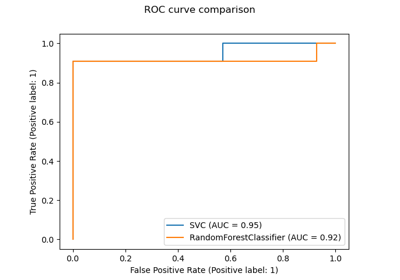Release Highlights for scikit-learn 0.22

Release Highlights for scikit-learn 0.22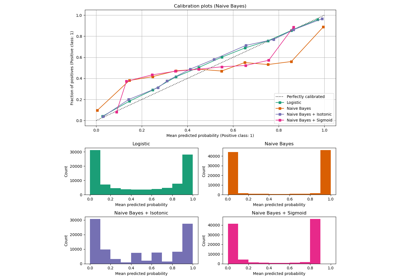Probability Calibration curves

Probability Calibration curves